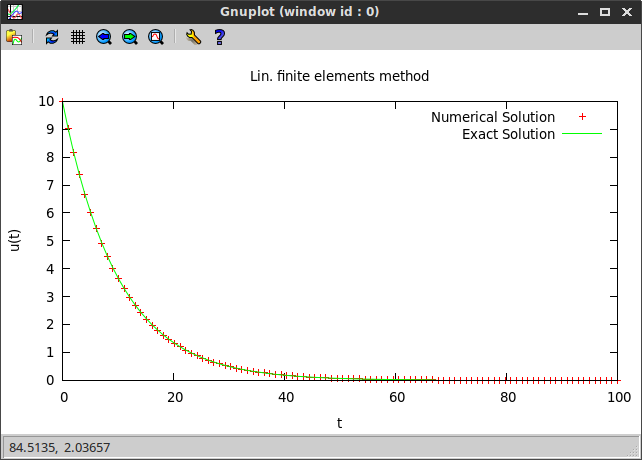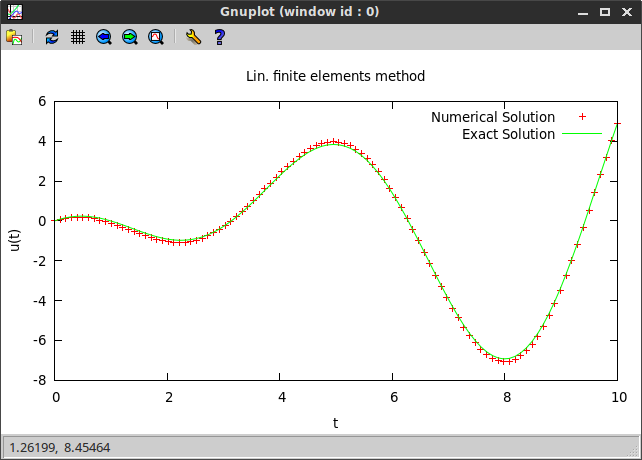# Solve two-point boundary value problems in Ruby with spitzy

A few days ago I programmed a numerical method for the solution for two-point boundary value problems, and today I discovered that I can use MathJax to display mathematical formulas in here (although there are some inconveniences related to the use of underscores). So, here goes another blog post!

### Background

Consider an ordinary differential equation of the following general form:

• ODE: $-(\alpha u’)’(x) + (\beta u’)(x) + (\gamma u)(x) = f(x)$, for $a < x < b$,
• where $\alpha$, $\beta$ and $\gamma$ are continuous functions of $x$ on $[a,b]$,
• with Dirichlet boundary conditions: $u(a) = u\subscript{a}$ and $u(b) = u\subscript{b}$.

We are looking for a weak solution to this problem, that is, a function $u$ that satisfies the integral equation

for every $v$ in the so-called test-function space $V$, which basically contains functions that have square-integrable distributional derivatives and vanish on the boundary of the domain of the ODE. If $u$ is a solution to the original formulation of the ODE then it also satisfies to integral formulation. However, a solution $u$ of the integral equation might not be twice differentiable.

#### Galerkin Method

The Galerkin method approximates $V$ with a finite dimensional functional space $V\subscript{h}$, which leads to a finite number of test functions $v$ that need to be tested against $u$ with the above integral equation. We denote the basis functions of $V\subscript{h}$ by $\phi\subscript{1}, \phi\subscript{2}, \ldots, \phi\subscript{N}$. Moreover, if we assume that $u$ also lies in the space $V\subscript{h}$, then the problem reduces to a linear system

where $A$ has entries

and the right hand side vector $\vec{f}$ has entries

The structure of $A$ and the degree of accuracy of the numerical solution depends on the form of the basis functions $\phi\subscript{1}, \phi\subscript{2}, \ldots, \phi\subscript{N}$, that is, on the choice of $V\subscript{h}$.

#### Finite Element Method

The finite element method chooses $V_h$ to be the space of continuous piecewise polynomials which are defined on subintervals of $[a, b]$ and vanish at $a$ and $b$.

Here, we only consider polynomials of degree 1, that is, continuous piecewise linear functions. Given an equally spaced grid $a = x\subscript{0} < x\subscript{1} < \ldots < x\subscript{n-1} < x\subscript{n} = b$, define for $i=1,2,\ldots,n-1$ the function $\phi\subscript{i}$ to be the continuous piecewise linear function that is equal to 1 at the node $x\subscript{i}$ and 0 at all other nodes. Thus, the basis functions have the following shape (image from a wikipedia article, the red line represents a linear combination of the basis functions):Finite element method 1D illustration2” by Krishnavedala - Own work. Licensed under CC BY-SA 3.0 via Wikimedia Commons.

### Implementation

I have implemented the linear finite element Galerkin method in class Bvp in Ruby for my project spitzy. It takes as inputs the interval of $x$ values as well as the Dirichlet boundary conditions at the two edges of the interval, the desired number of equally spaced grid points on which the numerical solution will be evaluated, and the functions $\alpha(x)$, $\beta(x)$, $\gamma(x)$ and $f(x)$ as Proc objects (or as Numeric if the function is constant).

The linear finite element Galerkin method boils down to a linear system $A\vec{u} = \vec{f}$, where the matrix $A$ is tridiagonal. Currently, my Ruby implementation uses the #solve method from the NMatrix gem. However, the memory usage as well as the speed of the algorithm can be improved, as soon as pull request #301, which implements a #solve_tridiagonal method, is merged into the project.

### Examples

Now let’s look at some examples.

#### Constant coefficients

First we look at example, where $\alpha$, $\beta$ and $\gamma$ are constants independent of $x$:

• ODE: $-800\pi u’’ + 8\pi u = 0$, $0 < x < 100$,
• BC: $u(0) = 10$, $u(100) = \frac{10}{\cosh(10)}$.

We compute the numerical solution:

require 'spitzy'
bvp_sol = Bvp.new(xrange: [0.0, 100.0], mx: 100, bc: [10.0, 0.00090799859],
a: 800.0*Math::PI, b: 0.0, c: 8.0*Math::PI, f: 0.0)


The exact solution is

We compare the obtained numerical to the exact solution by plotting both using the Ruby gnuplot gem:#### Non-constant coefficients

Now, let’s consider an example where $\alpha$, $\beta$, $\gamma$ and $f$ are all functions of $x$.

• ODE: $-\frac{d}{dx}(-\cos(x)u’(x)) + \sin(x)u’(x) + \cos(x) u(x) = -2\cos^2(x)$, $0 < x < 10$,
• BC: $u(0) = 0$, $u(10) = -9\sin(10)$.

We use spitzy to compute the numerical solution:

a = Proc.new { |x| -Math::cos(x) }
b = Proc.new { |x| Math::sin(x) }
c = Proc.new { |x| Math::cos(x) }
f = Proc.new { |x| -2.0*(Math::cos(x))**2 }
xrange = [0.0, 10.0]
bc = [0.0, (1.0 - 10.0) * Math::sin(10.0)]
bvp_sol = Bvp.new(xrange: xrange, mx: 100, bc: bc,
a: a, b: b, c: c, f: f)


By taking derivatives one can check that the exact solution is given by

Again, we plot both, the numerical and the exact solution, and observe that they approximately agree.Written on May 2, 2015